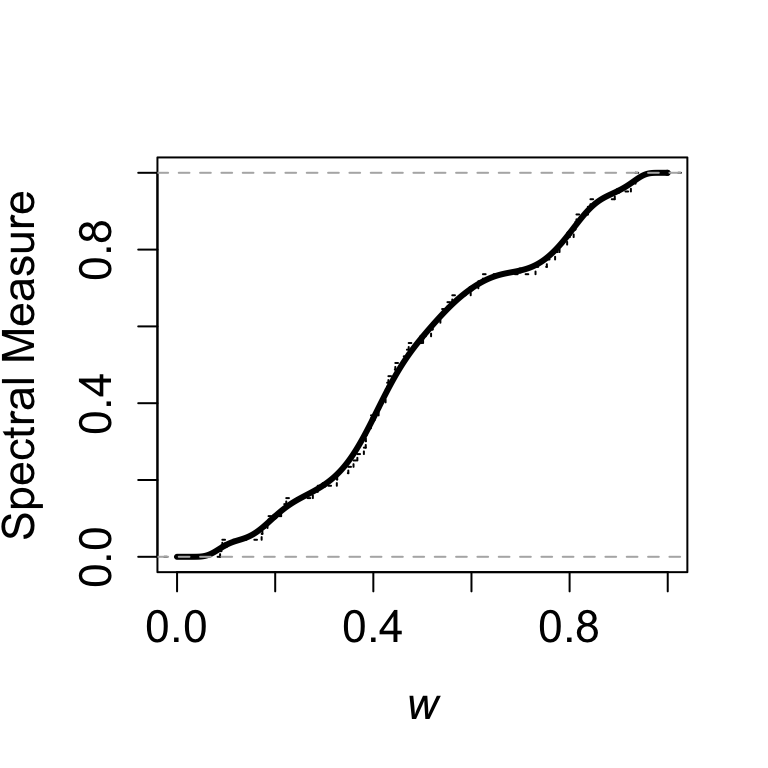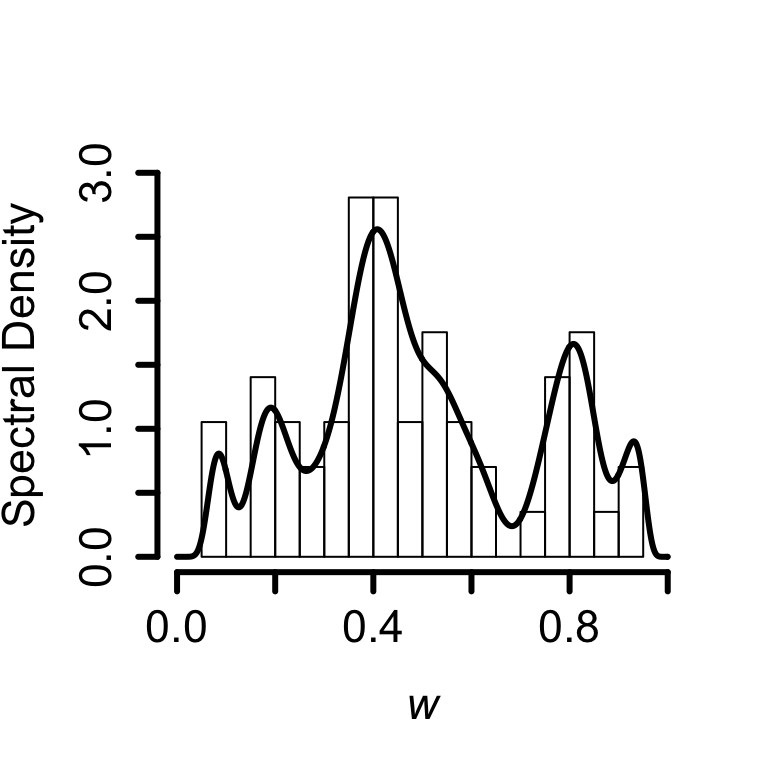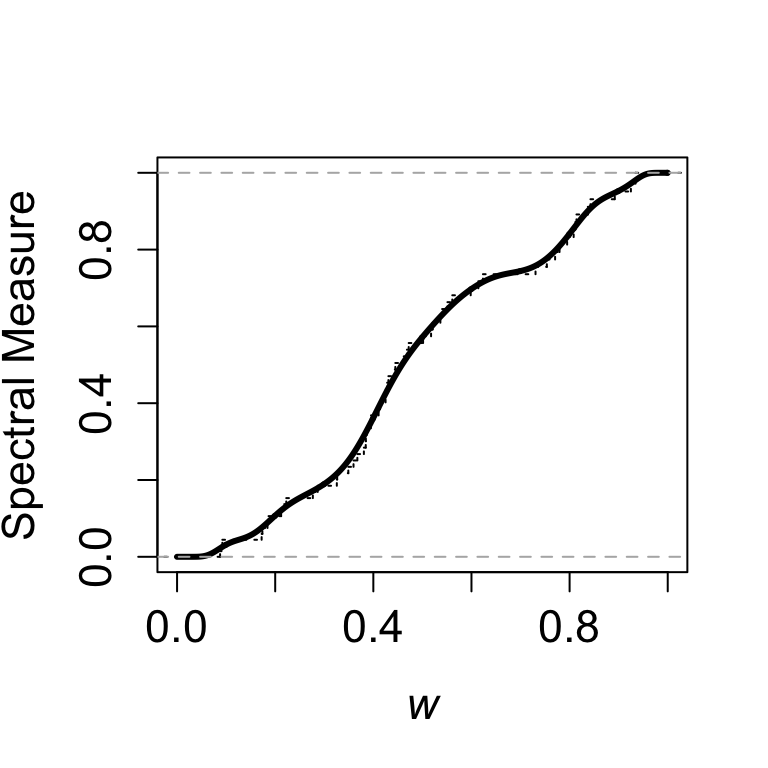### Smoothing methods for bivariate extremes

Here, I describe R code for fitting the maximum Euclidean likelihood spectral measures, as well as their corresponding smooth versions, and smooth spectral densities. The notation $$W_1,\dots, W_k$$ is here used to denote a sample of pseudo-angles, whose radius exceeds a large threshold $$\tau$$.

To compute the maximum Euclidean likelihood estimator one can use:

## maximum Euclidean likelihood estimator
eucdf <- function(w) {
w <- sort(w)
k <- length(w)
v <- var(w) * (k / (k - 1))
p <- 1 / k * (1 - (mean(w) - 1/2) * v^{-1} * (w - 1/2))
rval <- approxfun(w, cumsum(p), method = "constant", yleft = 0,
yright = 1, f = 0, ties = "ordered")
class(rval) <- c("ecdf", "stepfun", class(rval))
attr(rval, "call") <- sys.call()
rval
}

The code above has been designed to mimic that of R’s ecdf. The difference with respect to the empirical angular measures (Einmahl et al, 2001) is that the weights are no longer $$p_i = 1/k$$ but become pseudo-angle dependent, $$p_i = 1/k\{1 + (W_i - \overline{w})S^{-2}(W_i - 1/2)\}$$, so to enforce the moment constraint.

And how to construct smooth versions of maximum Euclidean likelihood spectral measure? This can be done by considering a version of kernel smoothing for the unit interval which still preserves the moment constraint. Details on the construction of such estimator can be found in de Carvalho et al (2013) and de Carvalho (2016). The code for fitting a smooth spectral measure is as follows:

seucdf <- function(w, nu, grid = seq(0, 1, .001)) {
k <- length(w)
lgrid <- length(grid)
v <- var(w) * ((k - 1) / k)
p <- 1 / k * (1 - (mean(w) - 1/2) * v^{-1} * (w - mean(w)))
ptilde <- numeric(k)
H <- numeric(lgrid)
for(i in 1:lgrid) {
for(j in 1:k) {
ptilde[j] <- p[j] * pbeta(grid[i], nu * w[j], nu * (1 - w[j]))
}
H[i] <- sum(ptilde)
}
outputs <- list(grid = grid, H = H, nu = nu, p = p)
}

Finally, to obtain the corresponding density, the code is essentially the same, but we need to replace the beta incomplete function (pbeta) with the density of a beta (dbeta), i.e.:

eudensity <- function(w, nu, grid = seq(0, 1, .001)) {
k <- length(w)
lgrid <- length(grid)
v <- var(w) * ((k - 1) / k)
p <- 1 / k * (1 - (mean(w) - 1/2) * v^{-1} * (w - mean(w)))
ptilde <- numeric(k)
h <- numeric(lgrid)
for(i in 1:lgrid) {
for(j in 1:k) {
ptilde[j] <- p[j] * dbeta(grid[i], nu * w[j], nu * (1 - w[j]))
}
h[i] <- sum(ptilde)
}
outputs <- list(grid = grid, h = h, nu = nu, p = p)
}

Analogously, the code for the Pickands dependence function is as follows:

spickands <- function(u, w, nu, grid = seq(0, 1, .001)) {
k <- length(w)
lgrid <- length(grid)
v <- var(w) * ((k - 1) / k)
p <- 1 / k * (1 - (mean(w) - 1/2) * v^{-1} * (w - mean(w)))
ptilde <- numeric(k)
A <- numeric(lgrid)
for(i in 1:lgrid) {
for(j in 1:k) {
ptilde[i] <- p[i] * as.numeric(integrate(integrand, 0, grid[i]))
}
A[i] <- 1 - u + 2 * sum(ptilde)
}
outputs <- list(grid = grid, A = A, nu = nu, p = p)
return(outputs)
}

There is a small typo on Eq. (13) of de Carvalho et al (2013) (there is an extra $$k$$).

### Revisiting extreme forest temperatures example

To illustrate the functions above, I will use data application from Ferrez et al (2011). The unit Fréchet transformed data can be downloaded from here. To compare the smoothed spectral measure (Euclidean weights) with the Euclidean spectral measure, I use the following code:

## Start by loading unit Fréchet transformed data
tau <- quantile(X + Y, 0.98)
grid <- seq(0, 1, .001)
w <- X / (X + Y)
w <- w[which(X + Y > tau)]
# bandwidth obtained from adapted cross-validation (Warchol, 2012)
nu <- 163
fit <- seucdf(w, nu)
# To check constraints type: (sum(p * fit$w)) plot(fit$grid, fit$H, type = "l", xlim = c(0,1), ylim = c(0,1), lwd = 3, xlab = expression(italic(w)), ylab = "Spectral Measure", cex.lab = 1.4, cex.axis = 1.4) lines(eucdf(w), verticals = TRUE, do.p = FALSE, lty = 3)Replicating Fig. 7 (left) in de Carvalho et al (2013) To confirm that the produced estimate obeys moment constraints type sum(fit$p * w)
##  0.5

To compute the smooth spectral density you will need to load the code from beta.density and then:

hist(w, breaks = 30, freq = F, xlim = c(0, 1), ylim = c(0, 3), lwd = 3,
xlab = expression(italic(w)), ylab = "Spectral Density", cex.lab = 1.4,
cex.axis = 1.4, main = "")
fit <- eudensity(w, nu)
lines(fit$grid, fit$h, type = "l", xlim = c(0,1),
ylim = c(0,2), lwd = 3)Replicating Fig. 7 (right) in de Carvalho et al (2013)

### Maximum empirical likelihood estimator and its smooth version

The maximum empirical likelihood estimator (MELE) of Einmahl and Segers (2009) can be implemented by using the function:

## maximum empirical likelihood estimator
elcdf <- function(w, delta) {
w <- sort(w) ;
k <- length(w)
p <- 1 / k * (1 + (w - 1/2) * delta)^-1
rval <- approxfun(w, cumsum(p), method = "constant", yleft = 0,
yright = 1, f = 0, ties = "ordered")
class(rval) <- c("ecdf", "stepfun", class(rval))
attr(rval, "call") <- sys.call()
rval
}

The difference with respect to the Euclidean spectral measure (eucdf) is that the empirical likelihood weights, $$p_i = 1/k \times 1\{(1 + \lambda (W_i - 1/2)\}$$, now depend on a Lagrange multiplier $$\lambda$$—which is responsible for enforcing the moment constraint; such Lagrange multiplier can be computed using the R package emplik. Despite these differences the Euclidean spectral measure and the empirical likelihood spectral measure are known to be asymptotically equivalent (cf de Carvalho et al, 2013, Thm 4.2)

Similarly as in the case of the Euclidean spectral measure, smoothed functionals of the empirical likelihood spectral measure can be constructed directly, and for instance the smoothed Empirical likelihood spectral measure can be computed using

selcdf <- function(w, nu, delta = delta, grid = seq(0, 1, .001)) {
k <- length(w)
lgrid <- length(grid)
v <- var(w) * ((k - 1) / k)
p <-  1 / k * (1 + (w - 1/2) * delta)^-1
ptilde <- numeric(k)
H <- numeric(lgrid)
for(i in 1:lgrid) {
for(j in 1:k) {
ptilde[j] <- p[j] * pbeta(grid[i], nu * w[j], nu * (1 - w[j]))
}
H[i] <- sum(ptilde)
}
outputs <- list(grid = grid, H = H, nu = nu, delta = delta, p = p)
}

Thus, if we want for instance to compare the smoothed spectral measure (Empirical likelihood weights) with the Empirical spectral measure, we can use the following code:

## Start by loading the emplik package and the unit Fréchet transformed data
require(emplik)
delta <- el.test(w, 1/2)$lambda fit <- selcdf(w, nu, delta) plot(fit$grid, fit$H, type = "l", xlim = c(0,1), ylim = c(0,1), lwd = 3, xlab = expression(italic(w)), ylab = "Spectral Measure", cex.lab = 1.4, cex.axis = 1.4) lines(eucdf(w), verticals = TRUE, do.p = FALSE, lty = 3)Replicating Fig.7 b in de Carvalho et al (2013) To confirm that the produced estimate obeys the moment constraint type sum(fit$p * w)
##  0.5